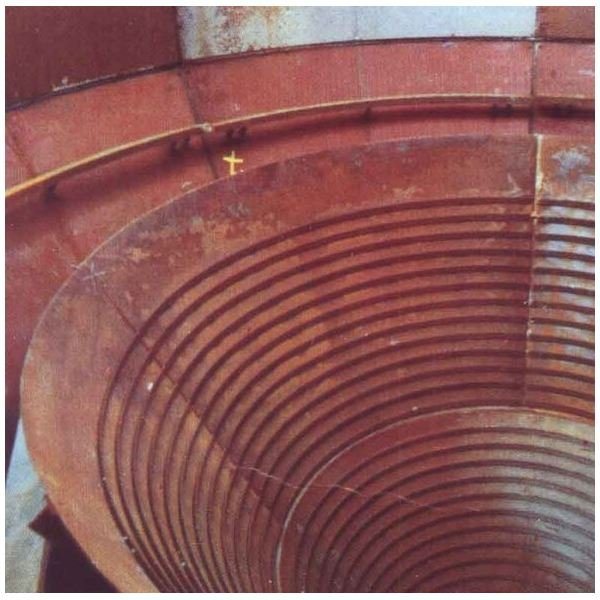Understanding How to Calculate the Material Burden Rate

DefinitionThe material burden rate, also known as the indirect production cost, is the ratio of overhead cost and an appropriate cost basis and finds widespread use to allocate overhead costs to products.

The manufacturing overhead costs include the cost of procurement of materials, administrative costs, cost of factory equipment, and packaging costs. The cost basis is labor, material, or space. For instance, the overhead or burden rate finds expression as a percentage of direct labor, or as the hourly cost rate based on machine hours.

Tax authorities and auditors usually monitor a company’s burden rate calculation to learn about the company's manufacturing overhead allocation, and through it, the operations and accounting practices.

Image Credit: Wikimedia Commons

Process

Material Burden Rate = Total Estimated Overhead Costs / Total Estimated Cost Drivers

The actual burden rate for a period reveals itself only after the accounting period is over. The industry practice is to forecast the anticipated burden rate for the next accounting period. The steps involved in the calculation are:

1. List all forecasted overhead expenses on materials for the accounting year, month wise. Use separate columns for each expense category such as material procurement cost, administrative cost, and other costs. Make sure to factor anticipated changes from present owing to change in orders, inflation, and other changes.
2. Total each column and all the sub-totals to find out the total estimated overhead costs.
3. Determine the total estimated cost driver. If the cost driver is labor, estimate the total labor units utilized during the period. If the cost driver is material, estimate the total production for the same period, and if the cost driver is space, ascertain the total area utilized during the period.
4. Divide the total estimated overhead costs by the total estimated cost driver to calculate material burden rate.

The best practice is to calculate material burden rates for each unit or division separately.

A computer spreadsheet best serves to calculate material burden rate. The calculation, however, is also doable using pen and paper.

Example

Assume a machine producing canisters produces 50 canisters a hour and runs 12 hours a day, six days a week. The total canister produced in a year is 50*12*6*52 weeks = 187,200 canisters.

Assume the total procurement cost of materials for the accounting period at \$25,000. Assume total administrative costs at \$5,000 per month, and packaging costs at \$2 per canister. Assume the depreciation of factory equipment at \$10,000 for the year.

Total material cost is (\$25,000) + (\$5,000 x 12 months) + (\$2 x 187,200 canisters) = \$25,000 + \$60,000+ \$374,400 = \$459,400

Calculate the material burden rate for 187,200 canisters by dividing the material cost by total number of canisters = \$459,400/187,200 = \$2.45. This means that to cover the material costs at the specified product schedule, each unit of canister should make \$2.45

The burden rate provides a complete picture of the costs associated with running a business. A high burden rate, for instance, explains why some things seemingly cheap to produce turn out very expensive.

References

1. New York State Society of CPA’s. “Glossary: Pretermined Overhead rate.” https://www.nysscpa.org/glossary Retrieved 11 December 2010.
2. West Virginia University. College of Business and Economics. “Cost Accumulation for Job/Shop and Batch Production Operations.” [DOC]. Retrieved from www.be.wvu.edu/divacctg/degeorge/…/Chapter3Lecture.doc on 11 December 2010.
3. Texas A&M ngineering. “Production Cost Models.” [PDF]. https://etidweb.tamu.edu/ftp/ENTC380/Exam%201%20Material/380%20Ch03b%20Production%20Costs.pdf. Retreived 11 December 2010.